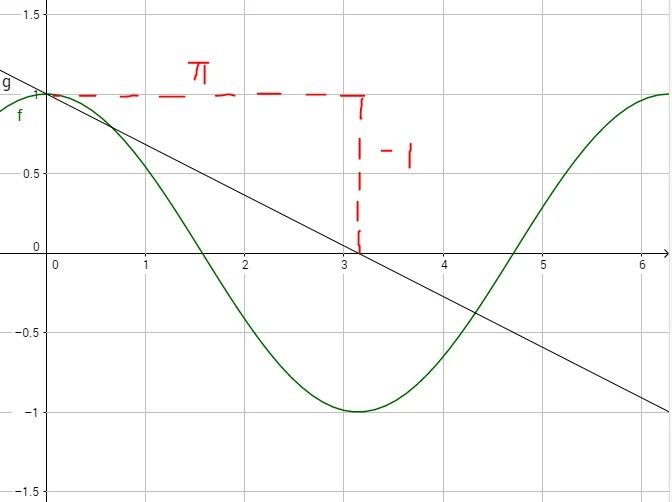The green curve represents the trigonometric curve y=cos x and the grey straight line represents$y=1-\frac{1}{\pi }x$The x-axis is from 0 to 2π (6.28) radian.

There are four points of intersection including the point (0,1) . Sometimes students do not include (0,1) because they either didn’t see the intersection at the left corner or they mistakenly believe that when two graphs touch  it is NOT an intersection.

Additional Math (amath) (A math)  Group Tuition. Woodlands Choa Chu Kang, Yew Tee, Yishun and Sembawang.